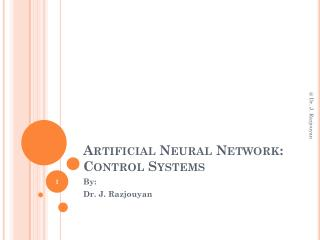DownloadDownload PresentationArtificial Neural Network: Control Systems

# Artificial Neural Network: Control Systems

Download Presentation## Artificial Neural Network: Control Systems

- - - - - - - - - - - - - - - - - - - - - - - - - - - E N D - - - - - - - - - - - - - - - - - - - - - - - - - - -
##### Presentation Transcript

1. Artificial Neural Network:Control Systems @ Dr. J. Razjouyan By: Dr. J. Razjouyan

2. Introduction • There are typically two steps involved when using neural networks for control: • System identification: In the system identification stage, you develop a neural network model of the plant that you want to control. • Control design: In the control design stage, you use the neural network plant model to design (or train) the controller. @ Dr. J. Razjouyan

3. Introduction • Three popular neural network architectures for prediction and control: • For model predictive control, the plant model is used to predict future behavior of the plant, and an optimization algorithm is used to select the control input that optimizes future performance. • For NARMA-L2 control, the controller is simply a rearrangement of the plant model. • For model reference control, the controller is a neural network that is trained to control a plant so that it follows a reference model. The neural network plant model is used to assist in the controller training. @ Dr. J. Razjouyan

4. NN Predictive Control • It uses a neural network model of a nonlinear plant to predict future plant performance. The controller then calculates the control input that will optimize plant performance over a specified future time horizon. • The first step in model predictive control is to determine the neural network plant model (system identification). • Next, the plant model is used by the controller to predict future performance. @ Dr. J. Razjouyan

5. NN Predictive Control:System Identification • The first stage of model predictive control is to train a neural network to represent the forward dynamics of the plant. The prediction error between the plant output and the neural network output is used as the neural network training signal. The process is represented by the following figure: @ Dr. J. Razjouyan

6. NN Predictive Control:System Identification • The neural network plant model uses previous inputs and previous plant outputs to predict future values of the plant output. The structure of the neural network plant model is given in the following figure. @ Dr. J. Razjouyan

7. NN Predictive Control:System Identification: Example • MATLAB • RUN THE DEMO predcstr @ Dr. J. Razjouyan